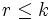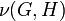Congruence condition on number of subgroups of given prime power order

This article describes a congruence condition on an enumeration, or a count. It says that in a finite group and modulo prime number, the number of subgroups of given prime power order satisfies a congruence condition.
View other congruence conditions | View divisor relations

Statement

Version for a group of prime power order

Let$G$ be a group of prime power order$p^k$ and suppose$0 \le r \le k$. The following are true:

• The number of subgroups of$G$ of order$p^r$ is congruent to$1$ modulo$p$.
• The number of normal subgroups of$G$ of order$p^r$ is congruent to 1 mod$p$.
• The number of p-core-automorphism-invariant subgroups of$G$ of order$p^r$ is congruent to 1 mod$p$.

In particular, the collection of groups of order$p^r$ is a collection of groups satisfying a universal congruence condition.

Version for a general finite group

Let$G$ be a finite group and$p^r$ be a prime power dividing the order of$G$. Then, the number of subgroups of$G$ of order$p^r$ is congruent to 1 mod$p$.

Proof

Equivalence between the multiple formulations

For a proof of the equivalence of the three formulations for a group of prime power order and the formulation for a general finite group, see collection of groups satisfying a universal congruence condition and equivalence of definitions of universal congruence condition.

Proof for a group of prime power order in terms of all subgroups using Facts (1) and (2)

The main advantage of this proof is that it has analogues for the congruence condition on number of subrings of given prime power order in nilpotent Lie ring.

This proof uses the principle of mathematical induction in a nontrivial way (i.e., it would be hard to write the proof clearly without explicitly using induction).

This proof uses fact (1): congruence condition relating number of subgroups in maximal subgroups and number of subgroups in the whole group, combined with an induction on order.

Given: A group$G$ of order$p^k$,$p$ a prime number.

To prove: For any$r \le k$, the number of subgroups in$G$ of order$p^r$ is congruent to$1$ modulo$p$.

Proof: In this proof, we induct on$k$, i.e., we assume the statement is true inside groups of order$p^l, l \le k$.

Base case for induction: The case$k = 0$ is obvious.

Inductive step: If$r = k$, the number of subgroups is 1, so the statement is true. So we consider$r < k$.

For a subgroup$H$ of$G$, denote by$n(H)$ the number of subgroups of$H$ of order$p^r$.

Step no. Assertion/construction Facts used Given data/assumptions used Previous steps used Explanation
1$n(G) \equiv \sum_{M \operatorname{max} G} n(M) \pmod p$. Here,$M \operatorname{max} G$ means that$M$ is a maximal subgroup of$G$. Fact (1)$r < k$ [SHOW MORE]
2 For every$M \operatorname{max} G$,$n(M) \equiv 1 \pmod p$. inductive hypothesis$r < k$ [SHOW MORE]
3 The number of maximal subgroups of$G$ is congruent to 1 mod$p$. Fact (2) Fact-direct.
4$n(G) \equiv 1 \pmod p$ Steps (1)-(3) [SHOW MORE]

Proof for a group of prime power order in terms of normal subgroups using Facts (3)-(5)

The main advantage of this proof is that it has analogues for the congruence condition on number of ideals of given prime power order in nilpotent Lie ring.

This proof uses the principle of mathematical induction in a nontrivial way (i.e., it would be hard to write the proof clearly without explicitly using induction).

This proof uses fact (3), combined with an induction on the order.

Given: A group$G$ of order$p^k$,$p$ a prime number.

To prove: For any$r \le k$, the number of normal subgroups in$G$ of order$p^r$ is congruent to$1$ modulo$p$.

Proof: In this proof, we induct on$k$, i.e., we assume the statement is true inside groups of order$p^l, l \le k$.

Base case for induction: The case$k = 0$ is obvious.

Inductive step: If$r = 0$, the number of normal subgroups is 1, so the statement is true. So we consider$r > 0$.

For a subgroup$H$ of$G$, denote by$\nu(G,H)$ the number of normal subgroups of$G$ of order$p^r$ containing$H$. Denote by$\nu(G)$ the total number of normal subgroups of$G$ of order$p^r$.

Step no. Assertion/construction Facts used Given data/assumptions used Previous steps used Explanation
1$\nu(G) \equiv \sum_N \nu(G,N) \pmod p$ where$N$ varies over all the minimal normal subgroups of$G$. Fact (3) [SHOW MORE]
2 For each minimal normal subgroup$N$ of$G$,$\nu(G,N)$ is congruent to 1 mod$p$. Fact (4) inductive hypothesis [SHOW MORE]
3 The number of minimal normal subgroups of$G$ is congruent to 1 mod$p$. Fact (5) Fact-direct.
4$\nu(G) \equiv 1 \pmod p$. Steps (1), (2), (3) [SHOW MORE]